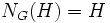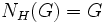# Self-normalizing subgroup

Names in other languages:British English: self-normalising subgroup
VIEW RELATED: Analogues of this | Variations of this | Opposites of this |
View a complete list of semi-basic definitions on this wiki
This article defines a subgroup property: a property that can be evaluated to true/false given a group and a subgroup thereof, invariant under subgroup equivalence. View a complete list of subgroup properties[SHOW MORE]

This is an opposite of normality

## History

The historical roots of this term, viz how the term and the concept were developed, are missing from this article. If you have any idea or knowledge, please contribute right now by editing this section. To learn more about what goes into the History section, click here

## Definition

### Symbol-free definition

A subgroup of a group is termed self-normalizing if it equals its own normalizer in the whole group.

### Definition with symbols

A subgroup$H$ of a group$G$ is termed self-normalizing if$N_G(H) = H$.

## Formalisms

BEWARE! This section of the article uses terminology local to the wiki, possibly without giving a full explanation of the terminology used (though efforts have been made to clarify terminology as much as possible within the particular context)

### First-order description

This subgroup property is a first-order subgroup property, viz., it has a first-order description in the theory of groups.
View a complete list of first-order subgroup properties

This is essentially because the normalizer of a subgroup has a first-order description.

### In terms of the in-normalizer operator

This property is obtained by applying the in-normalizer operator to the property: improper subgroup
View other properties obtained by applying the in-normalizer operator

## Metaproperties

### Transitivity

NO: This subgroup property is not transitive: a subgroup with this property in a subgroup with this property, need not have the property in the whole group
ABOUT THIS PROPERTY: View variations of this property that are transitive|View variations of this property that are not transitive
ABOUT TRANSITIVITY: View a complete list of subgroup properties that are not transitive|View facts related to transitivity of subgroup properties | View a survey article on disproving transitivity

A self-normalizing subgroup of a self-normalizing subgroup need not be self-normalizing.

Further information: Self-normalizing is not transitive

### Intermediate subgroup condition

YES: This subgroup property satisfies the intermediate subgroup condition: if a subgroup has the property in the whole group, it has the property in every intermediate subgroup.
ABOUT THIS PROPERTY: View variations of this property satisfying intermediate subgroup condition | View variations of this property not satisfying intermediate subgroup condition
ABOUT INTERMEDIATE SUBROUP CONDITION:View all properties satisfying intermediate subgroup condition | View facts about intermediate subgroup condition

Let$G \le H \le K$ be groups. Then the condition that$G$ is self-normalizing in$K$ means that$N_K(G) = G$ which will imply that$N_H(G) = G$, and hence that$G$ is self-normalizing in$H$.

Thus, any self-normalizing subgroup is also self-normalizing in every intermediate subgroup.

### NCI

This subgroup property is a NCI-subgroup property, i.e., it is identity-true subgroup property and further, the only normal subgroup of a group that satisfies the property is the whole group

It is clear that a subgroup that is both normal and self-normalizing must be the whole group -- that's because its normalizer equals both itself and the whole group.

### Intersection-closedness

This subgroup property is not intersection-closed, viz., it is not true that an intersection of subgroups with this property must have this property.
Read an article on methods to prove that a subgroup property is not intersection-closed

An intersection of self-normalizing subgroups need not be self-normalizing. This follows from the fact that it is a NCI-subgroup property, and hence cannot be normal core-closed.

### Join-closedness

This subgroup property is not join-closed, viz., it is not true that a join of subgroups with this property must have this property.
Read an article on methods to prove that a subgroup property is not join-closed

A join of self-normalizing subgroups need not be self-normalizing. This follows because the property of being self-normalizing is not normal closure-closed: there exist self-normalizing subgroups whose normal closure is a proper normal subgroup.

### Image condition

YES: This subgroup property satisfies the image condition, i.e., under any surjective homomorphism, the image of a subgroup satisfying the property also satisfies the property
View other subgroup properties satisfying image condition

If$H$ is a self-normalizing subgroup of$G$, and$f:G \to K$ is a surjective homomorphism of groups, then$f(H)$ is a self-normalizing subgroup of$K$.

Further information: Self-normalizing satisfies image condition

### Direct product-closedness

This subgroup property is direct product-closed: it is closed under taking arbitrary direct products of groups

If$H_1$ is a self-normalizing subgroup of math>G_1[/itex], and$H_2$ is a self-normalizing subgroup of$G_2$, then$H_1 \times H_2$ is a self-normalizing subgroup of$G_1 \times G_2$. The analogous statement holds for arbitrary direct products as well.

For full proof, refer: Self-normalizing is direct product-closed

## Effect of property operators

### The upward-closure

Applying the upward-closure to this property gives: weakly abnormal subgroup

If$H$ is a subgroup of$G$ such that every subgroup of$G$ containing$H$ is self-normalizing in$G$, then$H$ is termed a weakly abnormal subgroup of$G$. Being weakly abnormal is also equivalent to being contranormal in every intermediate subgroup.

## Testing

### GAP code

One can write code to test this subgroup property in GAP (Groups, Algorithms and Programming), though there is no direct command for it.
View the GAP code for testing this subgroup property at: IsSelfNormalizing
View other GAP-codable subgroup properties | View subgroup properties with in-built commands
GAP-codable subgroup property